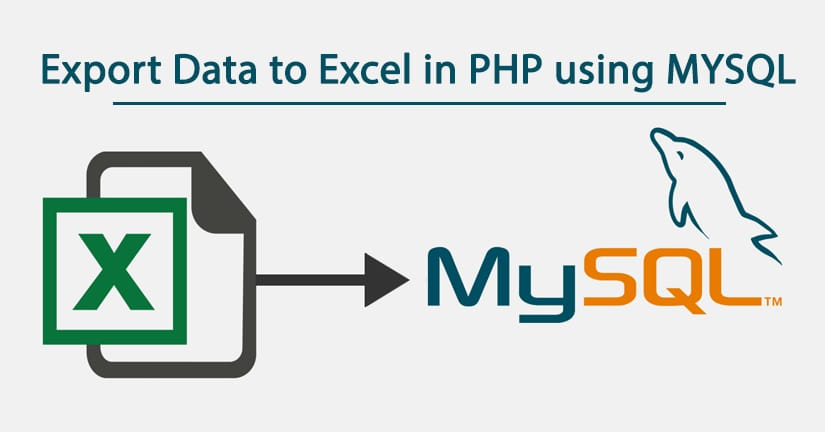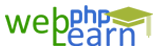Apply for Zend Framework Certification Training

# Corephp```<?php
define('LOCALHOST', 'localhost');
define('USER', 'root');
define('DATABASE', 'draw');
mysql_select_db(DATABASE);
\$select = "select * from tbl_zone_winner where tbl_zone_winner_status != ' '";
\$mq = mysql_query(\$select);
\$data ='';
while(\$data = mysql_fetch_assoc(\$mq)){
\$data_more[] = \$data;
}
//print_r(\$data_more);
//exit();
function filterData(&\$str)
{
\$str = preg_replace("/\t/", "\\t", \$str);
\$str = preg_replace("/\r?\n/", "\\n", \$str);
if(strstr(\$str, '"')) \$str = '"' . str_replace('"', '""', \$str) . '"';
}

\$fileName = "webphplearn_export_data" . date('Ymd') . ".xls";

\$flag = false;
foreach(\$data_more as \$row) {
if(!\$flag) {
// display column names as first row
echo implode("\t", array_keys(\$row)) . "\n";
\$flag = true;
}
// filter data
array_walk(\$row, 'filterData');
echo implode("\t", array_values(\$row)) . "\n";

}

exit;
?>                                    ```

`{{questionlistdata.blog_question_description}}`
`{{answer.blog_answer_description  }}`